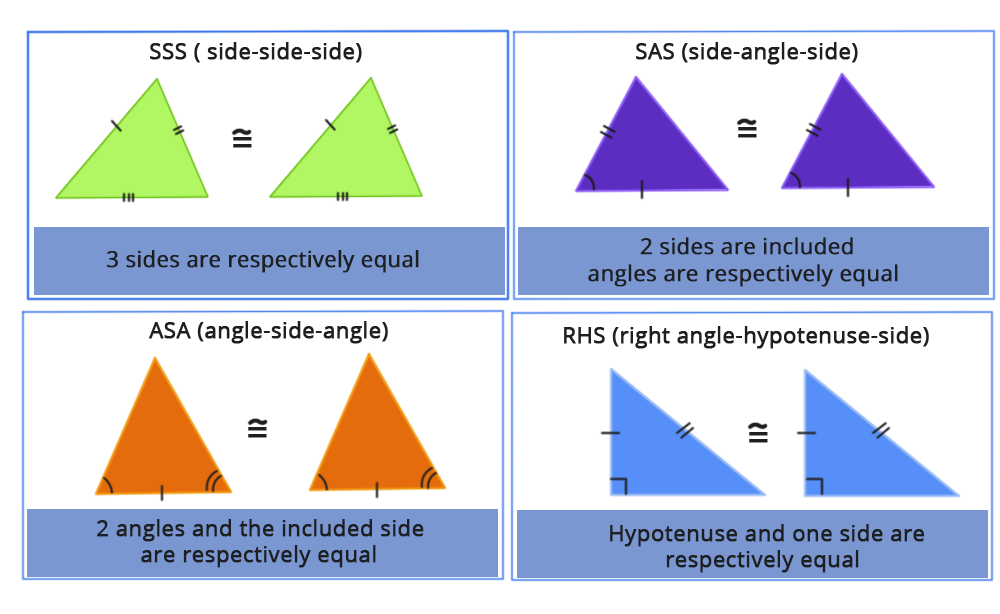• Call Now

1800-102-2727•

# Congruence of Triangles

Triangle congruence: Two triangles are said to be congruent if all three of their sides are equal and all three of their angles are equal in size. These triangles can be moved about, rotated, flipped, and turned to make them seem the same. They will line up if they are adjusted. Congruent triangles have the same sides and angles as each other. Four congruency criteria may be used to determine if two triangles are congruent. As a result, the congruence of triangles may be determined using only three of the six variables. In mathematics, congruence refers to the similarity of two figures depending on their shape and size.Meaning of Congruence

The term "congruence" is used to describe the relationship between an object and its mirror copy. When two items or forms superimposed on each other, they are said to be congruent. Their size and form are identical. When it comes to geometric figures, line segments of the same length and angles of the same measure are congruent.

In mathematics, the term congruent refers to figures and forms that may be moved or flipped to match the other ones. These shapes can be mirrored to match up with others.

Congruence in Triangles

When two shapes have the same shape and size, they are said to be congruent. If two forms are congruent, the mirror image of one of them is the same as the other.

CPCT in Triangles

A triangle is a polygon made up of three line segments that form three angles. When the sides of two triangles are the same length and the angles are the same measure, they are said to be congruent. Two triangles can be overlaid side by side and angle by angle in this manner.

When we study the congruency of triangles, we come across the word CPCT. The acronym CPCT stands for "Corresponding Parts of Congruent Triangles." The matching portions of congruent triangles are equal, as we know. We commonly utilize the abbreviation CPCT in brief terms instead of the complete version while dealing with triangle principles and solving problems.

SSS (Side-Side-Side)

The two triangles are said to be congruent if all three sides of one triangle are equal to the corresponding three sides of the second triangle.

SAS (Side-Angle-Side)

The two triangles are said to be congruent if any two sides and the angle included between the sides of one triangle are comparable to the corresponding two sides and the angle between the sides of the second triangle.

ASA (Angle-Side- Angle)

The two triangles are said to be congruent if any two angles and the side included between the angles of one triangle are comparable to the corresponding two angles and sides included between the angles of the second triangle.

AAS (Angle-Angle-Side)

Angle-angle-side is abbreviated as AAS. The triangles are said to be congruent when two angles and a non-included side of one triangle are identical to the corresponding angles and sides of another triangle.

RHS (Right angle- Hypotenuse-Side)

The two right triangles are said to be congruent if the hypotenuse and a side of one right-angled triangle are comparable to the hypotenuse and a side of the other right-angled triangle.

### What are Congruent Triangles and How Do You Use Them?

When the three sides and three angles of two triangles are equal in any orientation, they are said to be congruent.

### What does CPCT stand for in full?

Corresponding portions of Congruent triangles are abbreviated as CPCT. The CPCT theorem says that if two or more congruent triangles are selected, the corresponding angles and sides of the triangles are also congruent to each other.

### What are the Congruency Rules?

For triangles, there are five major laws of congruency:

• SSS Criterion that is Side-Side-Side
• SAS Criterion that is Side-Angle-Side
• ASA Criterion that is Angle-Side- Angle
• AAS Criterion that is Angle-Angle-Side
• RHS Criterion that is Right angle- Hypotenuse-SideTalk to our expert
Resend OTP Timer =
By submitting up, I agree to receive all the Whatsapp communication on my registered number and Aakash terms and conditions and privacy policy